../
• 2020-02-16
• Artificial Neural Networks

## Iranian License Plates

Automatic number-plate recognition (aka ANPR, ALPR, VLPR) for Iranian license plates. License plates are composed of digits 1 through 9 (not zero) and a subset of the Persian alphabet:

 1 = ۱ 2 = ۲ 3 = ۳ 4 = ۴ 5 = ۵ 6 = ۶ 7 = ۷ 8 = ۸ 9 = ۹ be = ب jim = ج dal = د sin = س sad = ص ta = ط qaf = ق lam = ل mim = م nun = ن vav = و h = ه or هـ ye = ی

Interesting trivia: after working on this project and marking up all those images of Iranian license plates, I now know my digits.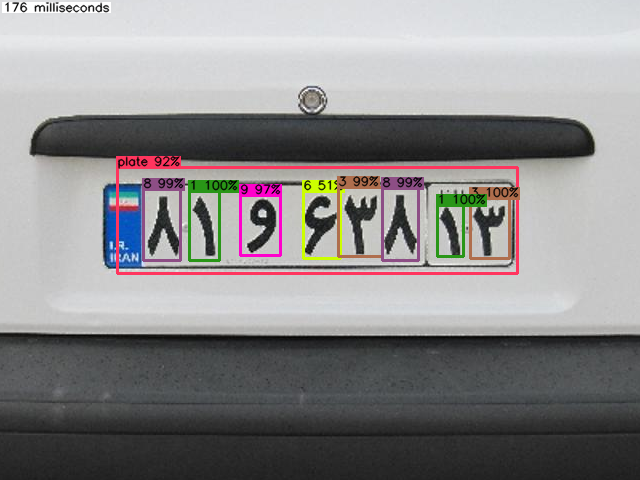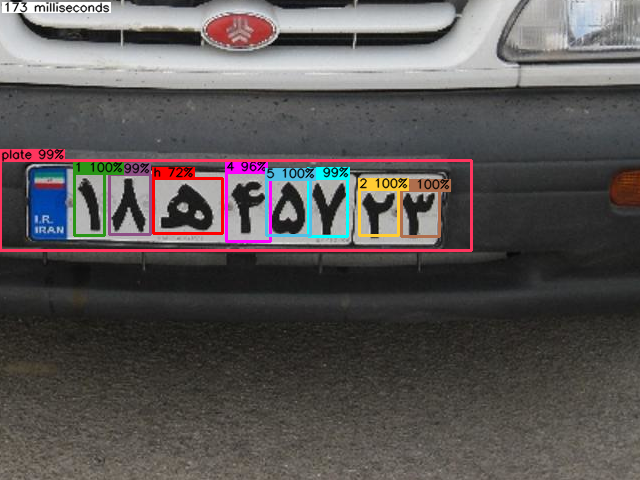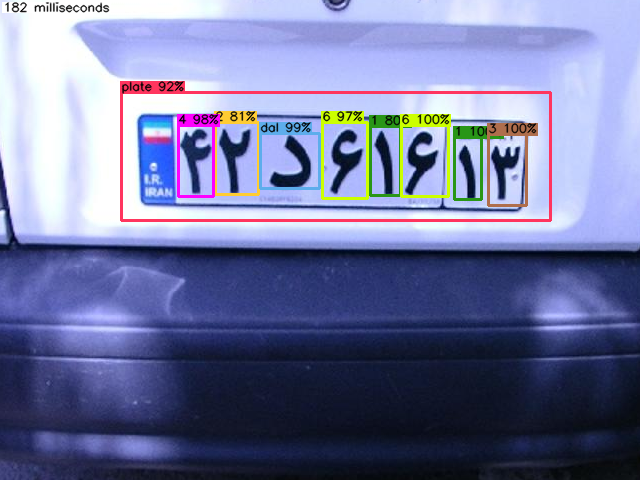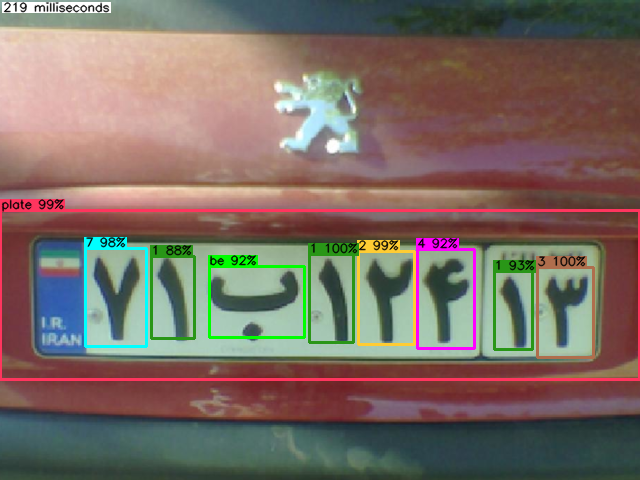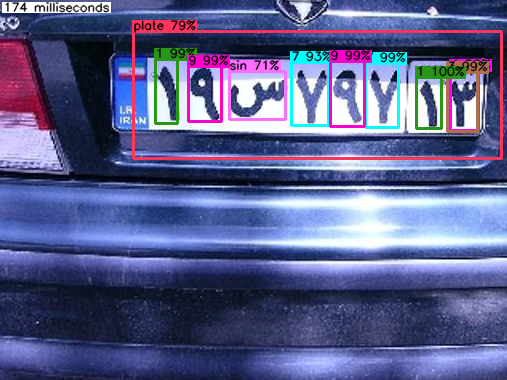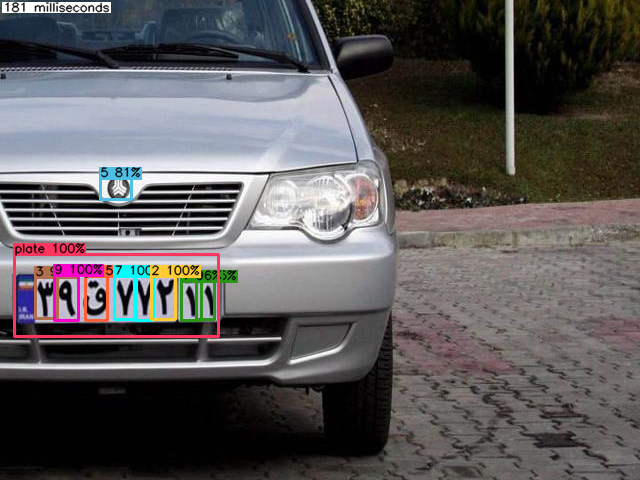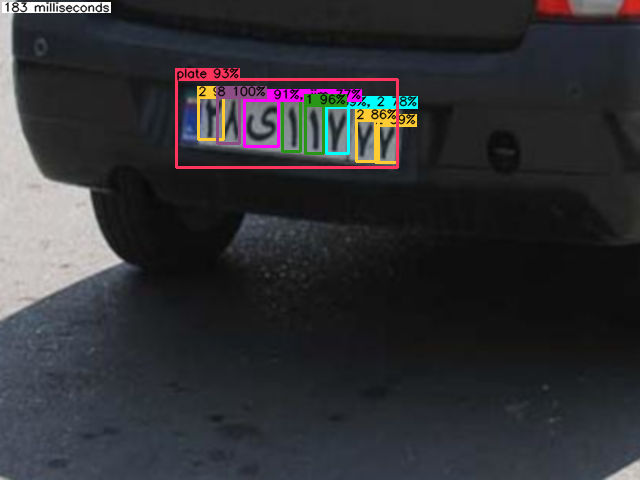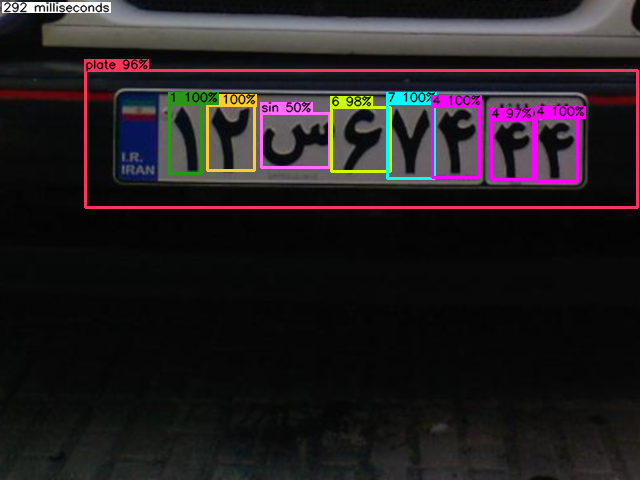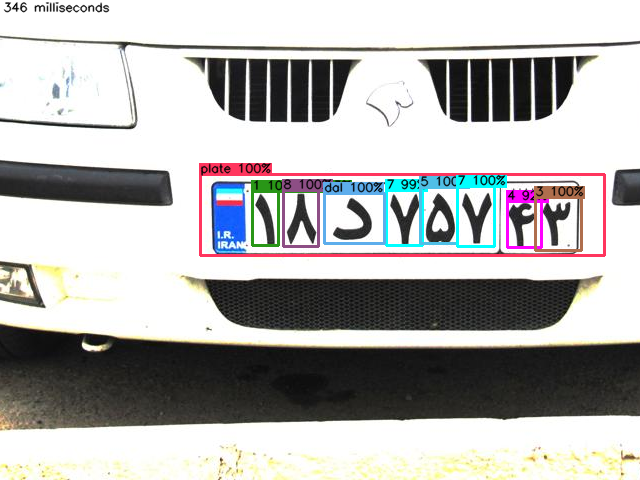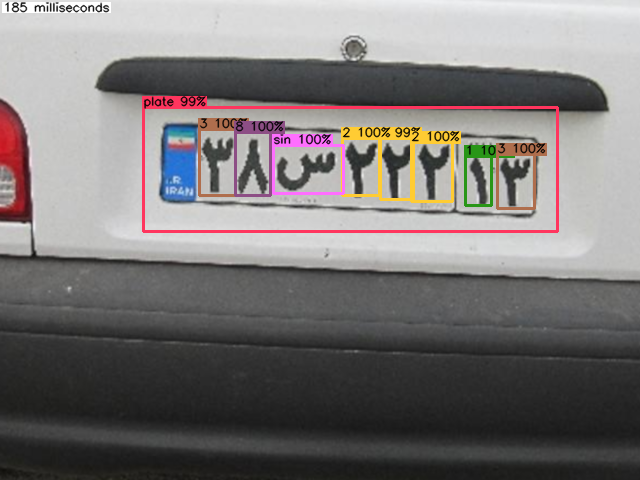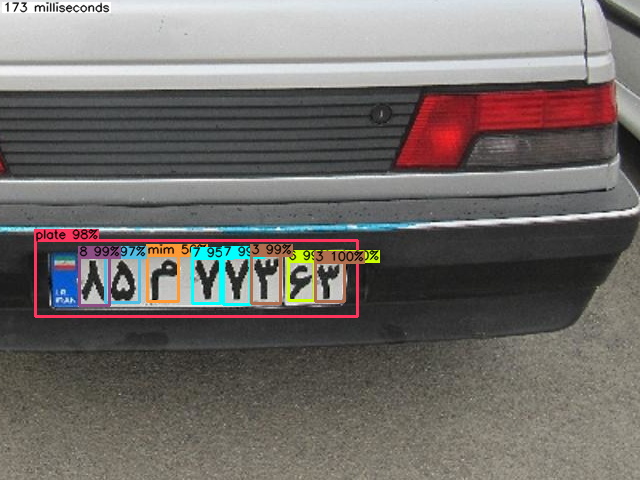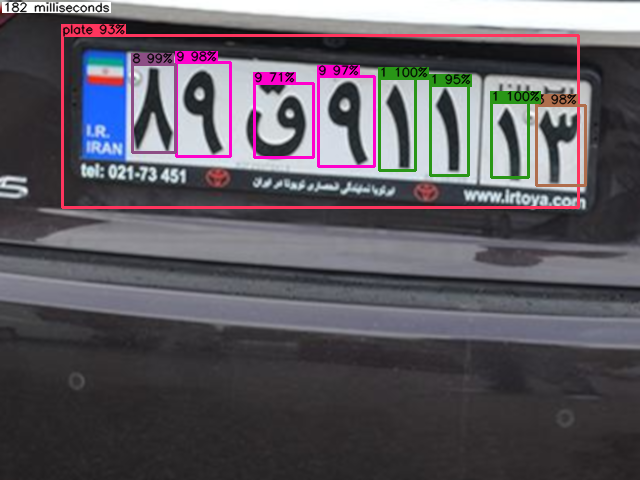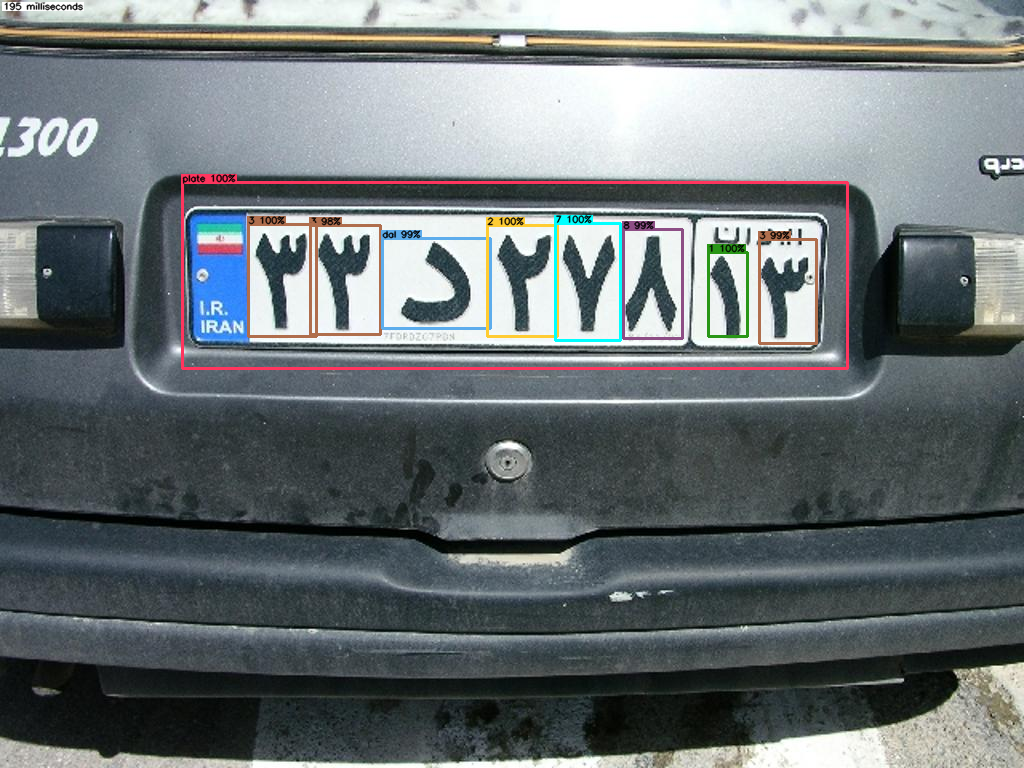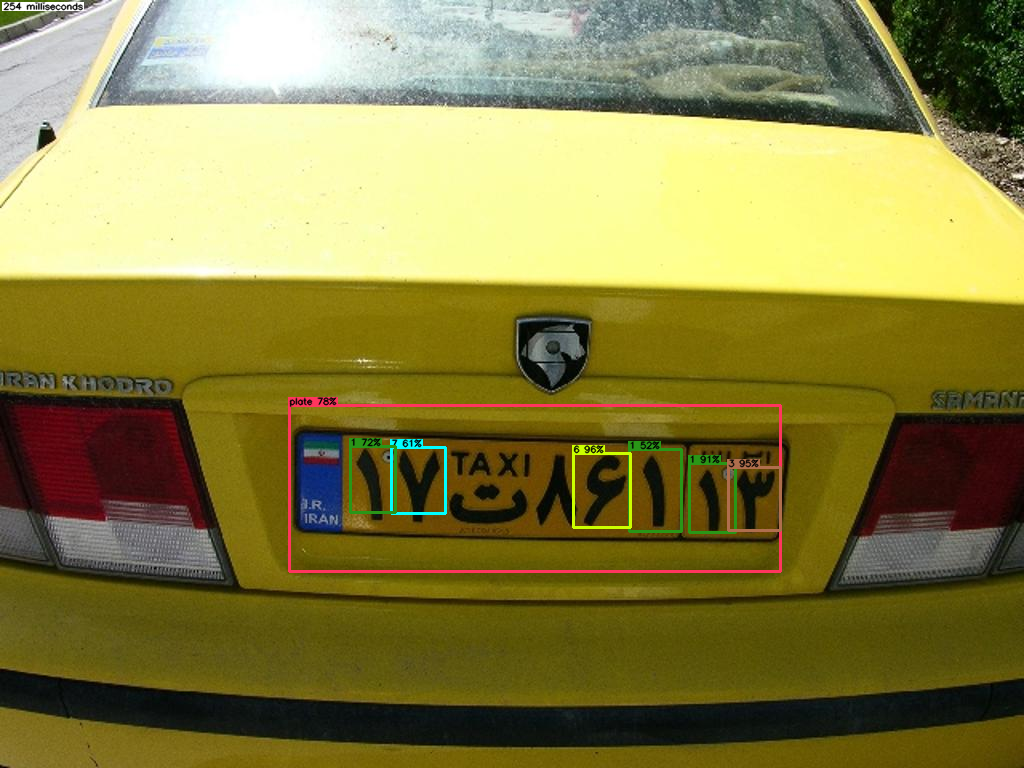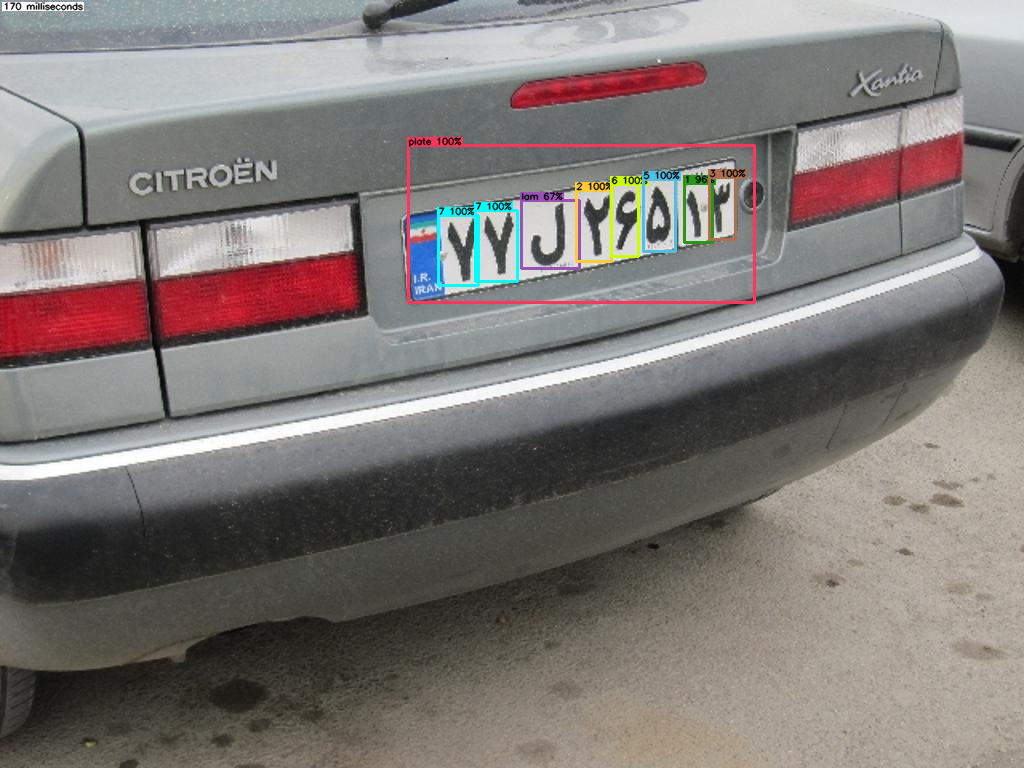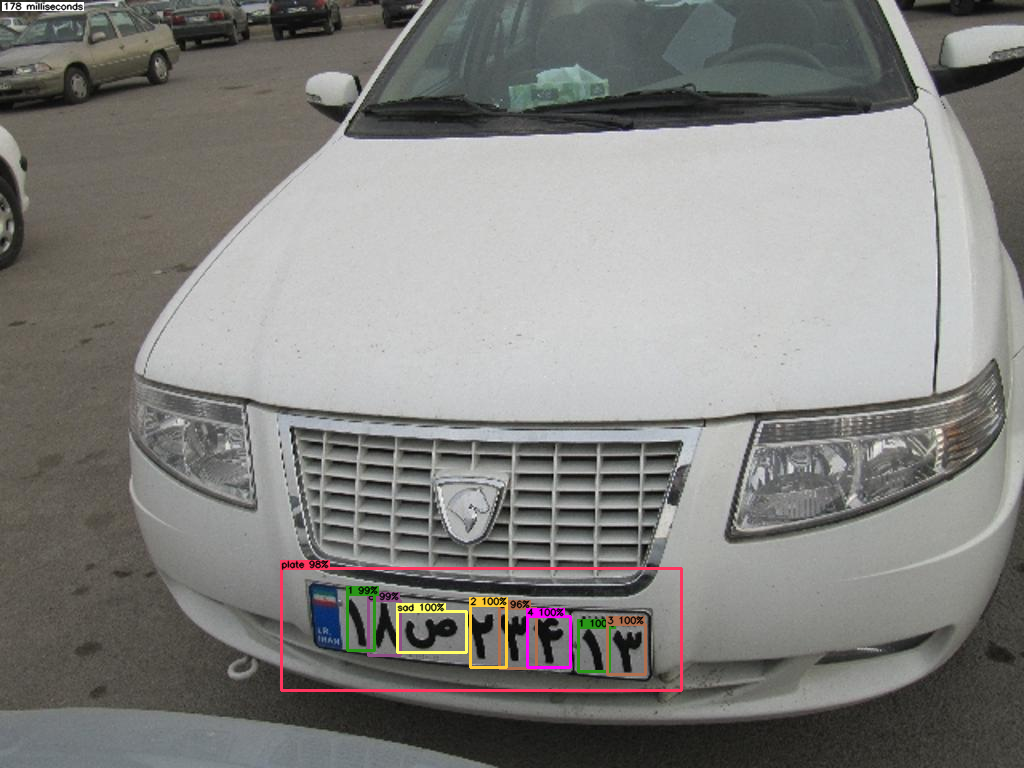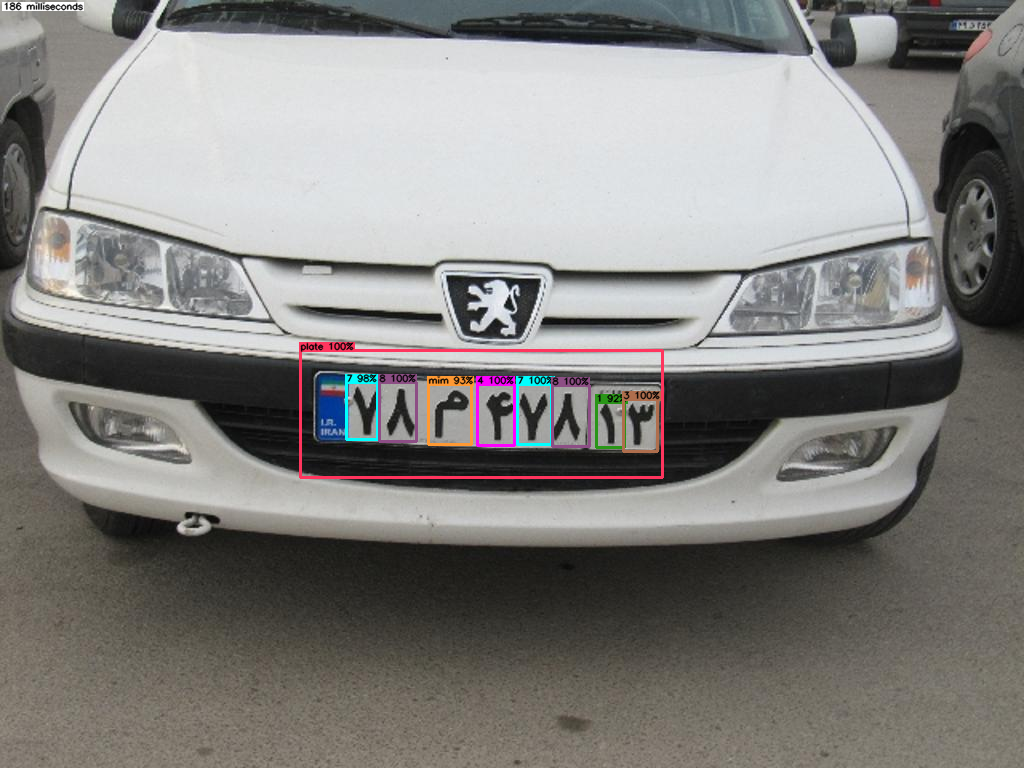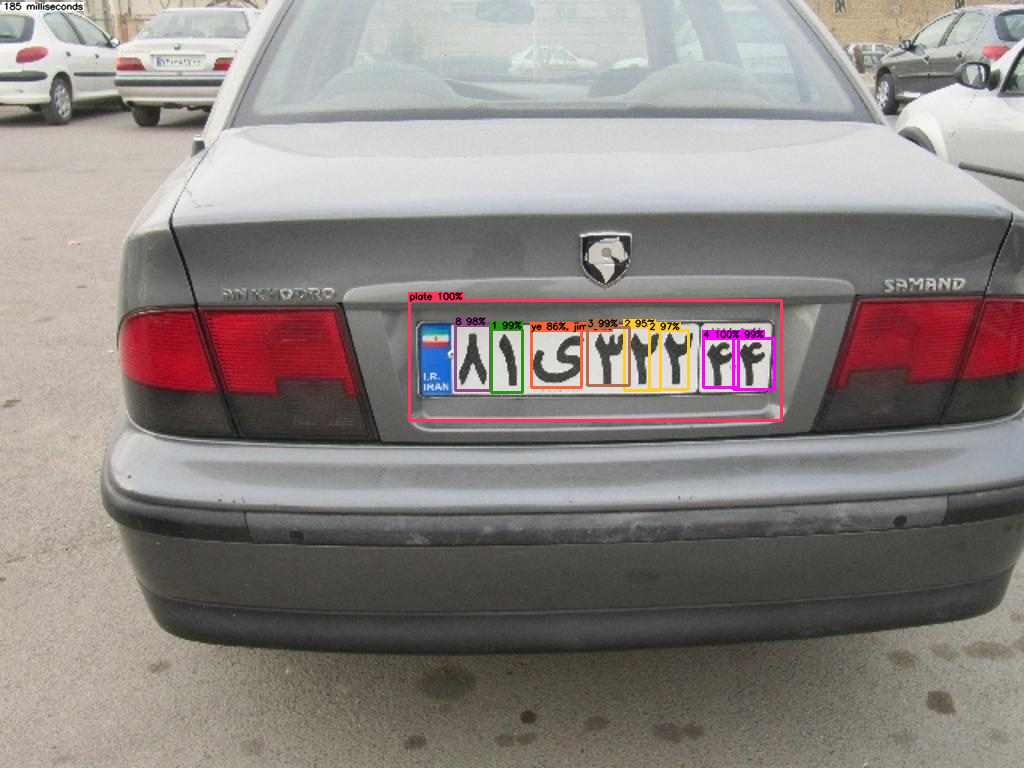../